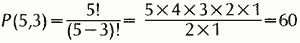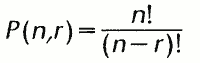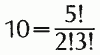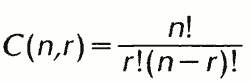probability

(redirected from likely)
Also found in: Dictionary, Thesaurus, Medical, Legal, Financial, Acronyms, Idioms, Wikipedia.

probability,

in mathematics, assignment of a number as a measure of the "chance" that a given event will occur. There are certain important restrictions on such a probability measure. In any experiment there are certain possible outcomes; the set of all possible outcomes is called the sample space of the experiment. To each element of the sample space (i.e., to each possible outcome) is assigned a probability measure between 0 and 1 inclusive (0 is sometimes described as corresponding to impossibility, 1 to certainty). Furthermore, the sum of the probability measures in the sample space must be 1.

Probability of Simple and Compound Events

A simple illustration of probability is given by the experiment of tossing a coin. The sample space consists of one of two outcomes—heads or tails. For a perfectly symmetrical coin, the likely assignment would be 1-2 for heads, 1-2 for tails. The probability measure of an event is sometimes defined as the ratio of the number of outcomes. Thus if weather records for July 1 over a period of 40 years show that the sun shone 32 out of 40 times on July 1, then one might assign a probability measure of 32/40 to the event that the sun shines on July 1.

Probability computed in this way is the basis of insurance calculations. If, out of a certain group of 1,000 persons who were 25 years old in 1900, 150 of them lived to be 65, then the ratio 150/1,000 is assigned as the probability that a 25-year-old person will live to be 65 (the probability of such a person's not living to be 65 is 850/1,000, since the sum of these two measures must be 1). Such a probability statement is of course true only for a group of people very similar to the original group. However, by basing such life-expectation figures on very large groups of people and by constantly revising the figures as new data are obtained, values can be found that will be valid for most large groups of people and under most conditions of life.

In addition to the probability of simple events, probabilities of compound events can be computed. If, for example, A and B represent two independent events, the probability that both A and B will occur is given by the product of their separate probabilities. The probability that either of the two events A and B will occur is given by the sum of their separate probabilities minus the probability that they will both occur. Thus if the probability that a certain man will live to be 70 is 0.5, and the probability that his wife will live to be 70 is 0.6, the probability that they will both live to be 70 is 0.5×0.6=0.3, and the probability that either the man or his wife will reach 70 is 0.5+0.6−0.3=0.8.

Permutations and Combinations

In many probability problems, sophisticated counting techniques must be used; usually this involves determining the number of permutations or combinations. The number of permutations of a set is the number of different ways in which the elements of the set can be arranged (or ordered). A set of 5 books in a row can be arranged in 120 ways, or 5×4×3×2×1=5!=120 (the symbol 5!, denoting the product of the integers from 1 to 5, is called factorial 5). If, from the five books, only three at a time are used, then the number of permutations is 60, orIn general the number of permutations of n things taken r at a time is given byOn the other hand, the number of combinations of 3 books that can be selected from 5 books refers simply to the number of different selections without regard to order. The number in this case is 10:In general, the number of combinations of n things taken r at a time isStatistical Inference

The application of probability is fundamental to the building of statistical forms out of data derived from samples (see statisticsstatistics,
science of collecting and classifying a group of facts according to their relative number and determining certain values that represent characteristics of the group.
). Such samples are chosen by predetermined and arbitrary selection of related variables and arbitrary selection of intervals for sampling; these establish the degree of freedom. Many courses are given in statistical method. Elementary probability considers only finite sample spaces; advanced probability by use of calculus studies infinite sample spaces. The theory of probability was first developed (c.1654) by Blaise Pascal, and its history since then involves the contributions of many of the world's great mathematicians.

Bibliography

See P. Billingsley, Probability and Measure (1979); I. Hacking, The Emergence of Probability (1984, rev. ed. 2006); J. T. Baskin, Probability (1986); P. Bremaud, Introduction to Probability (1988); S. M. Ross, Introduction to Probability Theory (1989).

probability

(STATISTICS) a number ranging from 0 (impossible) to 1 (certain) that indicates how likely it is that a specific outcome will occur in the long run. Probability theory is concerned with setting up a list of rules for manipulating probabilities and calculating the probabilities of complex events. It predicts how random variables are likely to behave and provides a numerical estimate of that prediction. In sociology, it is particularly important for sampling procedures and statistical inference. See also EXPLANATION. A probability sample is another name for a RANDOM SAMPLE, i.e. a sample selected in such a way that all units in the population have a known chance of selection. The advantage of using random (probability) samples in sociological research is that probability theory enables an estimate to be made of the amount of sampling error when sample results are generalized to the population. see SAMPLE AND SAMPLING.

Statistical inference deals with two related problems, the estimation of unknown population parameters and the testing of hypotheses from sample data. From sample data summary descriptive statistics are obtained, for example, the sample mean or the sample proportion. The Central Limit Theorem states that if random large samples of equal size are repeatedly drawn from any population, sample statistics such as the mean will have a normal (Gaussian) distribution. One property of this distribution is that there is a constant proportion of probabilities lying within a specified distance of the mean. It is this characteristic that allows statistical inferences from random sample statistics to populations. Calculating a sample mean does not allow a certain statement of what the population mean is, but with the knowledge of the sampling distribution an estimate of it can be made with a specific level of confidence, e.g. a probability of 0.95 (95%), or 0.99 (99%).

A SIGNIFICANCE TEST tests the probability of an observed result in sample data occurring by chance. Knowledge of the theoretical frequency distributions allows a probability value to be attached to the test statistic and if this is sufficiently low, e.g. p≤0.05 or p≤0.O1, the NULL HYPOTHESIS is rejected (see SIGNIFICANCE TESTS). Both tests of significance and confidence levels are based on the laws of probability.

Probability

in mathematics, a numerical characteristic of the degree of possibility of the occurrence of some specific event under one or another specific condition repeatable an unlimited number of times. As a category of scientific knowledge, the concept of probability reflects a particular type of connection between phenomena that are characteristic of large-scale processes. The category of probability forms the basis of a particular class of regularities—probability or statistical regularities.

The numerical value of a probability in certain cases is obtained from the classical definition of probability: the probability is equal to the ratio of the number of favorable cases of a given event to the number of equally possible cases. For example: if a given city has distributed 500,000 bonds out of 10 million bonds of the state lottery-loan, from which one prize of maximum size must come out in a single drawing, the probability that the maximum prize will fall to an inhabitant of this city is equal to 500,000/10,000,000 = 1/20.

In other, more complex cases, the determination of the numerical value of a probability requires a statistical approach. For example, if in 100 trials a rifleman hits a target 39 times, then one can suppose that the probability of his hitting the target under the given conditions is approximately equal to 4/10. Using a probability obtained in the classical or statistical manner, one can calculate new probabilities in accordance with the rules of the theory of probability. For example, if for our rifleman, the probability of a hit for a single shot is 4/10, then the probability that he will make at least one hit in four shots is equal to 1 - (1 - 4/10)4 ≈ 0.87. This conclusion can be verified statistically: if attempts to strike the target with at least one shot in four are repeated many times, then they will succeed in approximately 87 percent of the cases (on the assumption that within this time the rifleman’s skill does not change appreciably).

Mathematical probability is an expression of the qualitatively distinctive connection between the random and the necessary. In the exposition of probability theory, those features of probability which at a given stage of scientific development were necessary for such development are formulated in the form of axioms. However, neither these axioms, nor the classical approach to probability, nor the statistical approach give an exhaustive definition of the real substance of the concept of probability; they are merely known approximations to its ever more complete disclosure. By far not every event, the occurrence of which for given conditions is not uniquely determined, has for this complex of conditions a definite probability. The assumption that under given conditions and for a given event, a probability, that is, a completely determined fraction of the number of occurrences of a given event for a large number of repetitions of given conditions, exists, is a hypothesis that each individual problem requires a special proof or basis. For example, it makes sense to speak of the probability of hitting a target of given dimensions from a given distance with a rifle of a certain model by a rifleman picked at random from a specific military division. However, it would be meaningless to speak of the probability of hitting a target if nothing is known about the shooting conditions.

Apropos of the connection of probability with frequency, it is necessary to bear in mind the following: for a finite number n repetitions of specific conditions, the fraction of m cases in which the given event occurs, that is, the so-called frequency m/n, as a rule, differs little from the probability p. The larger the number of n repetitions, the more rarely one encounters any significant deviation of the frequency m\n from the probability p. To explain this circumstance, let us consider the example of coin tossing in which the probability of a “head” and “tail” is identical and equal to 1/2. For ten tosses (n = 10), the occurrence of ten heads or ten tails is only slightly probable. But to assert that heads will come out exactly five times is also without sufficient basis, and, what is more, in asserting that a head comes out four, five, or six times, we still run a great risk of being wrong. But, for 100 tosses, it is possible without appreciable risk to assert in advance that the number of heads will be between 40 and 60.

Mathematical probability can serve as an estimate of the probability of an event in the ordinary, everyday sense, that is, to define so-called problematical judgments that are usually expressed by such words as “possibly,” “probably,” and “very likely.” Apropos of these estimates, one must bear in mind that in applying them to any specific judgment, which, in fact, can be only true or false, the estimate of its probability has only provisional or subjective meaning, that is, it expresses only our relationship to the matter. For example, if someone not having special information as regards this matter wants to imagine the condition of the environs of Moscow on Mar. 23, 1930, then he will say: “Probably, on this day there was snow on the fields.” However, in fact, in 1930 the snow in the Moscow area was already gone from the fields by March 22. Having ascertained this situation, we must revoke the original estimate that was expressed by the problematical judgment enclosed in quotation marks. Nevertheless, this estimate, which was found to be erroneous when applied to the given individual case, is based on the reliable general rule: “At the beginning of the fourth week of March the fields near Moscow are, for the most part, covered with snow.” This rule reflects the objective properties of the Moscow area climate. This kind of rule can be expressed by indicating the level of probability of the event in question under one or another general condition realizable an unlimited number of times. These estimates now have an objective meaning. Consequently, the use of a calculus of probability for the corroboration of our estimates of the degree of reliability of one or another assertion relating to separate individual events must not give rise to the opinion that mathematical probability is only the numerical expression of our subjective certainty in the occurrence of a certain event. Such an idealistic, subjective understanding of the meaning of mathematical probability is erroneous. When developed logically, it leads to the absurd assertion that out of pure ignorance, by analyzing only the subjective states of our greater or lesser certainty, we can draw any definite conclusion with regard to the external world.

The use of the calculus of probability described above to estimate a situation in separate individual cases inevitably leads to the question of what kinds of probabilities can be ignored in practice. This question is resolved in different ways depending on how great the necessity of a rapid passage from the accumulation of reliable data to their effective use is. For example, if under given shooting conditions the theoretical calculation reduces to the fact that the combat problem posed will be solved by a given number of shots with the probability 0.95 (that is, the probability that the designated number of projectiles will not suffice is 0.05), then it is usually considered possible, in directing combat operations, to proceed on the assumption that the designated number of projectiles will prove to be sufficient. In the more tranquil conditions of scientific investigations, it is customary to disregard only a probability of 0.003 (this norm is connected with the so-called three-sigma rule), and that sometimes one can require an even further approximation of the probability, in the absence of error, to one. In mathematical statistics, a probability that is being disregarded in a given investigation is called a significance level. Although in statistics it is usually recommended to use significance levels of 0.05 in preliminary crude investigations and of 0.001 in final serious inferences, considerably greater reliability in probability conclusions is often attained. For example, the fundamental conclusions of statistical physics are based on disregarding only a probability of the order of less than 0.0000000001.

REFERENCES

Matematika, ee soderzhanie, melody i znachenie, vol. 2, ch. 11. Moscow, 1956.
Kolmogorov, A. N. “K logicheskim osnovam teorii informatsii i teorii veroiatnostei.” In the collection Problemy peredachi informatsii, vol. 5, part 3. Moscow, 1969.

A. N. KOLMOGOROV

probability

[‚präb·ə′bil·əd·ē]
(statistics)
The probability of an event is the ratio of the number of times it occurs to the large number of trials that take place; the mathematical model of probability is a positive measure which gives the measure of the space the value 1.
References in periodicals archive ?
This is a considerable reduction from 2016, when 63 percent of residents believed that a terrorist attack in the country was either ''likely'' or ''very likely''.
The second half of June is likely to remain wet in which temperatures are likely to drop by
Due to this rainfall, the rivers like Brahmaputra and its tributaries in Arunachal Pradesh, Assam and Meghalaya, Northern tributaries of Ganga in Eastern UP, North Bihar are likely to Rise and create low to High flood with unprecedented flood in few locations.
Least likely: To not be fawned over by all the ladies in the camp.
A full 80% of respondents said a candidate being Jewish makes them no more or less likely to vote for him, 10% said they were less likely to vote for a Jew for president, and 8% said they were more likely.
Some of you are likely to resume your exercise regimen to come back in shape.
They are also less likely to experience premature birth compared to other types of care, including that led by GPs or consultants.
Weatherman Alex Zadnik predicted thunderstorms would likely occur during the day, that is likely to have maximum of 25 degree temperature.
Ironically for merchants, 40% of consumers said they were either somewhat likely (19%) very likely (10%) or extremely likely (11%) to use credit cards instead.
They were also 42% more likely to report feeling depressive sadness, 55% more likely to have been in a physical fight during the past year, 3.4-fold more likely to have ever had sex, and 88% more likely to have had four or more sexual partners.
They were also 2.7-fold more likely to rarely or never wear a bike helmet and 39% more likely to rarely or never wear a seat belt, reported Dr.

Site: Follow: Share:
Open / Close